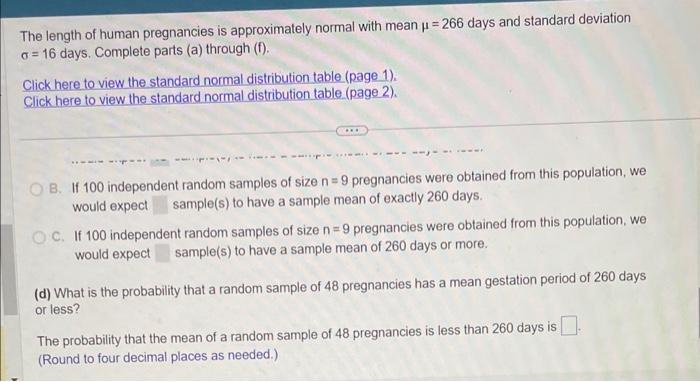Home / Expert Answers / Statistics and Probability / the-length-of-human-pregnancies-is-approximately-normal-with-mean-266-days-and-standard-deviatio-pa379

# (Solved): The length of human pregnancies is approximately normal with mean =266 days and standard deviatio ...The length of human pregnancies is approximately normal with mean days and standard deviation days. Complete parts (a) through (f). Click here to view the standard normal distribution table (page 1). Click here to view the standard normal distribution table (page 2). B. If 100 independent random samples of size pregnancies were obtained from this population, we would expect sample(s) to have a sample mean of exactly 260 days. C. If 100 independent random samples of size pregnancies were obtained from this population, we would expect sample(s) to have a sample mean of 260 days or more. (d) What is the probability that a random sample of 48 pregnancies has a mean gestation period of 260 days or less? The probability that the mean of a random sample of 48 pregnancies is less than 260 days is (Round to four decimal places as needed.)

We have an Answer from Expert